## # 1 Introduction

### # 什么是并行计算

• 并行计算 (parallel computing)：在同一个计算机体系的多个处理器/电脑一起工作解决一个问题
• 分布式计算 (distributed computing)：分布在多个计算机体系（异地）的处理器/电脑一起工作解决一个问题
• 并行计算机 (parallel computer)：一个多核的计算机系统
• 并行计算机分为多计算机系统和中心化多处理器
• 多计算机系统 (multicomputer)：多个计算机通过内部网络连接
• 中心化多处理器 (centralized/symmetrical multiprocessor, SMP)：一个计算机系统，其中所有 CPU 共享一个全局内存

### # 为什么需要并行计算

• 微处理器性能增长越来越慢
• 同样的性能下，并行系统功耗更低

### # 并行计算关心的问题

• 架构上的问题：
• Pipline, ILP...
• 缓存一致性
• 单共享总线 or 网络
• UMA, NUMA, CC-NUMA, Cluster...
• 编程模型上的问题：
• 单寻址空间 or 多寻址空间
• 进程使用锁、消息传递 or 其他方法进行同步
• 分布式 or 中心化内存
• 故障可靠性
• 性能表现上的问题：
• 指标：规模、加速比、可扩展性
• Models: PRAM，BSP，PPRAM...
• 并行计算的评价方法
• 其他问题：
• 编程语言
• 编程工具
• 可移植性
• Automatic programming of parallel computers
• Education of parallel computing philosophy

### # 如何编写并行程序

• 需要明确告诉不同处理器如何分工
• 需要把串行程序改写为并行
• 有时候直接改写的效率非常低，需要设计全新的算法

#### # 举个栗子

sum = 0;
for (i = 0; i < n; i++)
{
x = compute_next_value(...);
sum += x;
}


my_sum = 0;
my_first_i = ...;
my_last_i = ...;
for (my_i = my_first_i; my_i < my_last_i; my_i++)
{
my_x = compute_next_value(...);
my_sum += my_x;
}


if (I am the master core) {
sum = my_x;
for each core other than myself {
sum += value;
}
} else {
send my_x to the master;
}


Work with odd and even numbered pairs of cores. Pair the cores so that core 0 adds its result with core 1's result，Core 2 adds its result with core 3's result, etc. Repeat the process now with only the evenly ranked cores. Core 0 adds result from core 2. Core 4 adds the result from core 6, etc. Now cores divisible by 4 repeat the process, and so forth, until core 0 has the final result.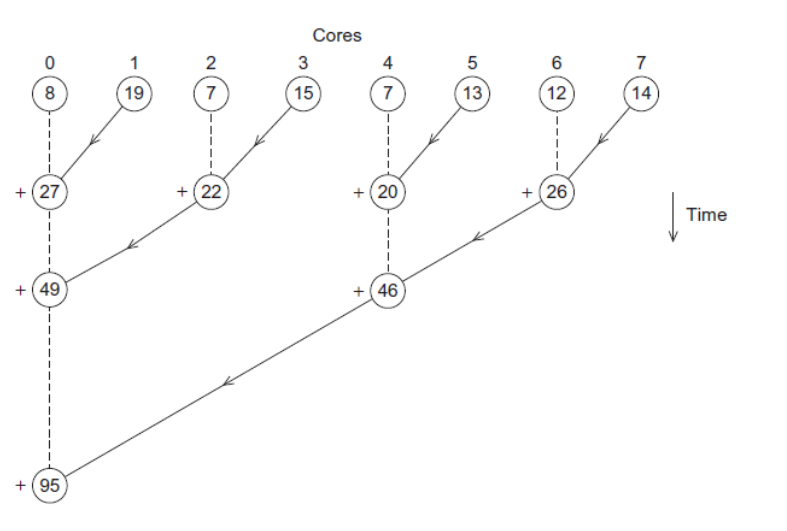#### # 并行程序的编写方向

1. 任务并行：将整个任务分成很多不同的小任务
2. 数据并行：将数据进行分块，每个核在自己分到的数据上做相似的任务

• 任务并行（将试卷按题目进行划分）：A 老师批改 1-5 题，B 老师批改 6-10 题，C 老师批改 11-15 题
• 数据并行（将试卷按张数进行划分）：每位老师各批改 100 张试卷

### # 习题

（这个题目后面应该会讲）

\begin{aligned} my_first_i &= \lfloor\frac{i*n}{p}\rfloor \\ my_last_i &= \lfloor\frac{(i+1)*n}{p}\rfloor - 1 \end{aligned}

my_last_i 比较好理解，因为任务分配是连续的，my_last_i 就是 下一个核的 my_first_i 减 1。

3 3 4 3 3 4 3 3 4


## # 2 Parallel Programming Platform

### # 对冯诺依曼体系的改进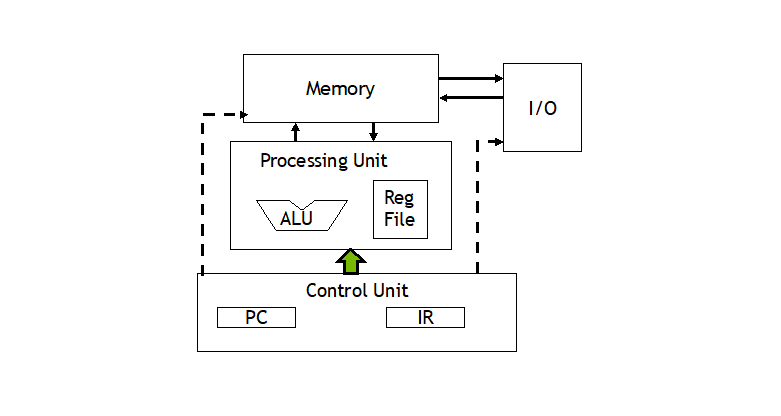1. 缓存
2. 虚拟存储
3. 底层的并行（指令集并行、线程级并行）

#### # 缓存Cache 命中（Cache hit）和不命中（Cache miss）

##### # 缓存的写策略

1. Write-through（写直达）：更新缓存的同时更新主存；缓存和主存始终一致，但每次写缓存的速度会变慢。
2. Write-back（写回）：更新缓存时标记缓存为脏数据（dirty），当该行缓存被替换时，脏数据会被写回至主存；写缓存的速度不受影响，但缓存替换时的速度会变慢。
##### # 缓存的映射方法
1. 全映射：每行内存可以映射在缓存的任意位置
2. 直接映射：每行内存只能映射在缓存的固定位置（一般是内存行数下标 % 缓存总行数）
3. n 路组相联映射：将缓存每 n 行分为一组，每行内存可以映射在缓存的固定的组的 n 行中任意一行（一般组号 = 内存行数下标 % 缓存总行数 % n）

##### # 缓存优化的技巧

1. 合并数组（data merge）：通过将两个独立数组合并为一个复合元素的数组来改进空间局部性
2. 循环交换（loop interchange）：通过改变循环嵌套来按序访问存储器中存储的数据
3. 循环合并（loop fusion）：将两个具有相同循环类型且有一些变量重叠的独立循环合并
4. 块化（blocking）：通过不断使用一些数据块（而不是完整地遍历一行和一列）来改进时间局部性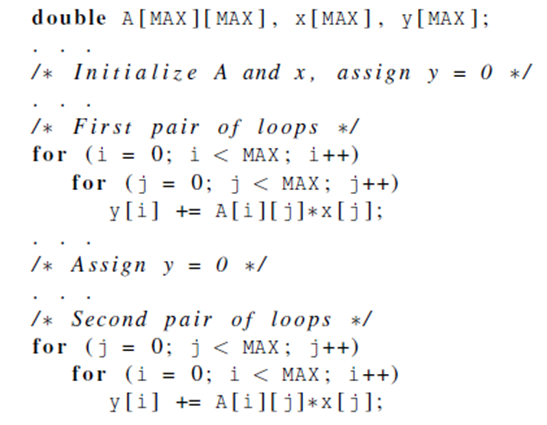#### # 虚拟内存

##### # 指令级并行
1. 流水线技术，参考计算机系统结构
2. 某些情况下，多条指令也可以被同时发射
3. 分支预测

### # 并行计算的硬件

1. SISD（传统冯诺依曼模型）
2. SIMD：对多个数据进行相同操作，1 个控制单元 + 多个 ALU
3. MISD（尚未开发）
4. MIMD：使用多个指令流同时操作多个数据流，多个独立操作单元 + 各自的 ALU

#### # MIMD 物理组织

1. 共享缓存架构（Shared Cache Architecture），多为单芯片多处理器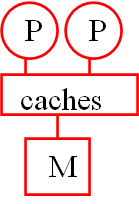1. 统一内存寻址（Uniform Memory Access，UMA）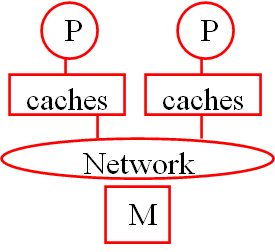1. 独立内存寻址（Non-Uniform Memory Access，NUMA）

NUMA 并不是处理器完全不能访问其他块的内存，而是处理器可以直接访问一部分内存+通过处理器内置的特殊硬件访问其他内存。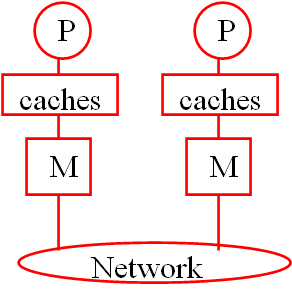1. 分布式系统/内存、集群（Distributed System/Memory）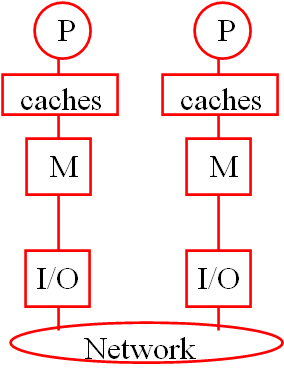#### # 多维 Mesh 网络

Mesh：将一维线性的网络拓展到二维、三维或更高维度，结点之间只能和邻居进行交流。

#### # 超立方体结构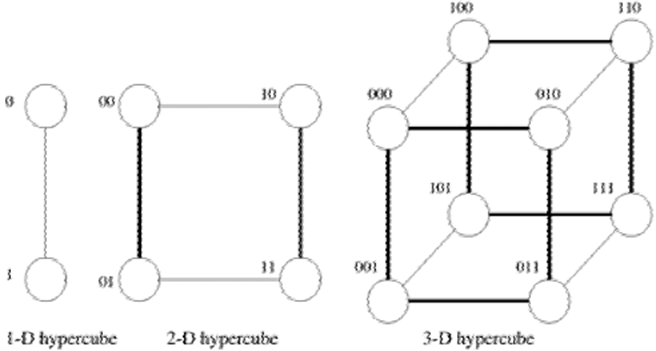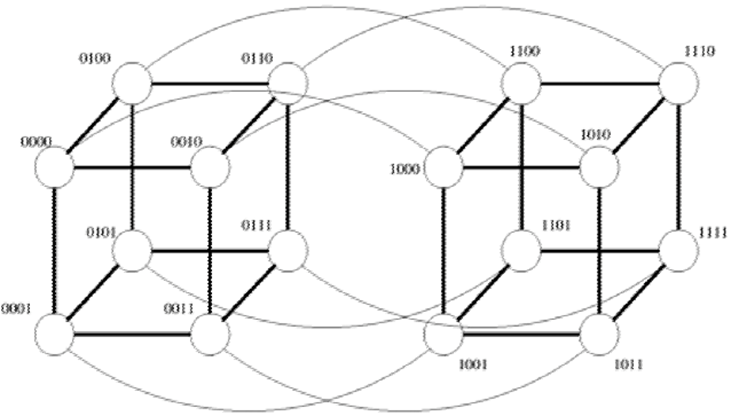#### # 缓存一致性• Write Invalidate：处理器写自己的缓存时，使其他缓存失效；Write-through 下还需要更新内存，Write-back 下需要使内存失效。
• Write Update：处理器写自己的缓存时，立即更新其他缓存；Write-through 下还需要更新内存，Write-back 下需要标记缓存为脏，在缓存失效的时候写回内存。

##### # 写失效协议

• Shared：存在多份有效的数据（写会导致其他失效）
• Modified：只有当前数据有效（写不会导致其他失效）
• Invalidate：数据无效（读会请求数据）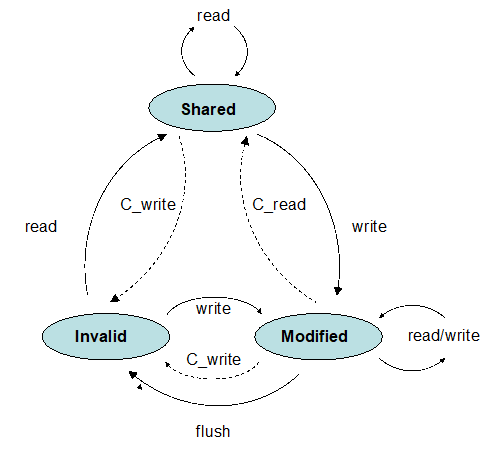1. 当一个数据是 Modified 后，所有操作都直接在本地进行，无需向外部广播。
2. 多个核读入一个数据时，所有缓存的内容都会变为 Shared，随后所有的读操作都直接在本地进行，无需向外部广播。
3. 多个核同时读和写时，会出现（在带宽上的）瓶颈
##### # 基于目录的缓存一致性协议
• 基于目录：共享的状态都存储在（位于内存的）“目录”
• 目录里用一位表示 shared/dirty 状态（State）
• 目录里用一个 bitmap 表示数据被缓存在哪些处理器（Presence Bits）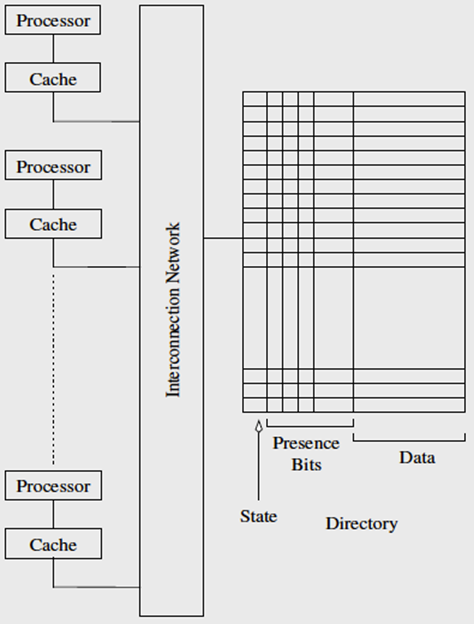1. 处理器 0 和处理器 1 读 x，此时状态为 shared，0 和 1 的 presence bits 均为 1
2. 处理器 0 写变量，状态变为 dirty，1 的 presence bits 为 0
3. 处理器 2 读变量，将会请求处理器 0 写回，随后 0 和 2 的 presence bits 均为 1

### # 并行计算的软件

• 内存共享系统上，一个进程 fork 出多个线程
• 分布式系统上，需要多个进程

SPMD: single program multiple data，MPI 和 CUDA 都是用的都是这种。

SPMD 的写法：

char message [ 1 0 0 ] ;
. . .
my_rank = Get_rank ( ) ;
if ( my_rank == 1) {
sprintf ( message , "Greetings from process 1" ) ;
Send ( message , MSG_CHAR , 100 , 0 ) ;
} else if ( my_rank == 0) {
Receive ( message , MSG_CHAR , 100 , 1 ) ;
printf ( "Process 0 > Received: %s\n" , message ) ;
}


## # 3 Parallel Program Design

### # Foster 四步走

• Partitioning：分块
• Communication：通信
• Agglomeration：组合
• Mapping：映射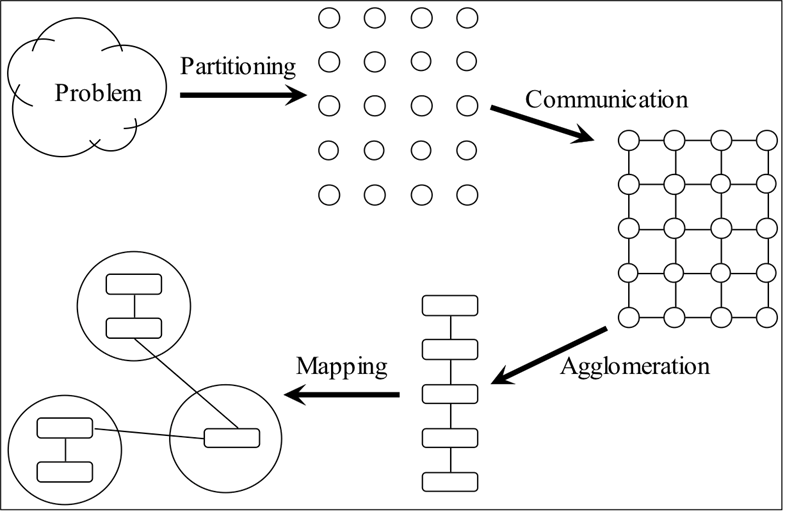### # 分块

Domain vs. Functional Decomposition

### # 通信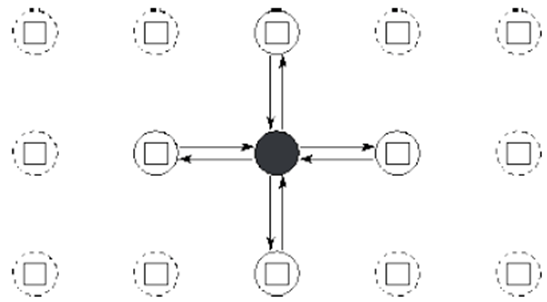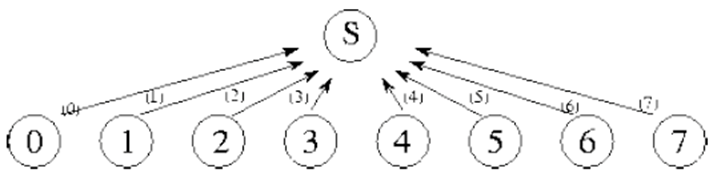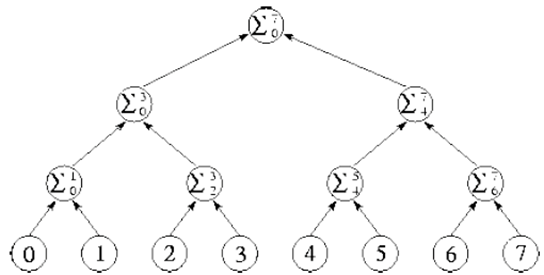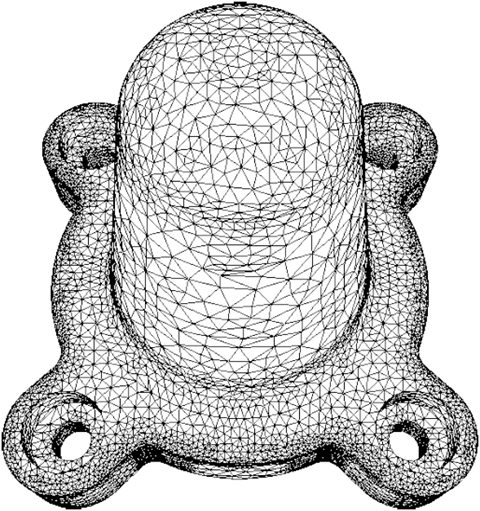### # 映射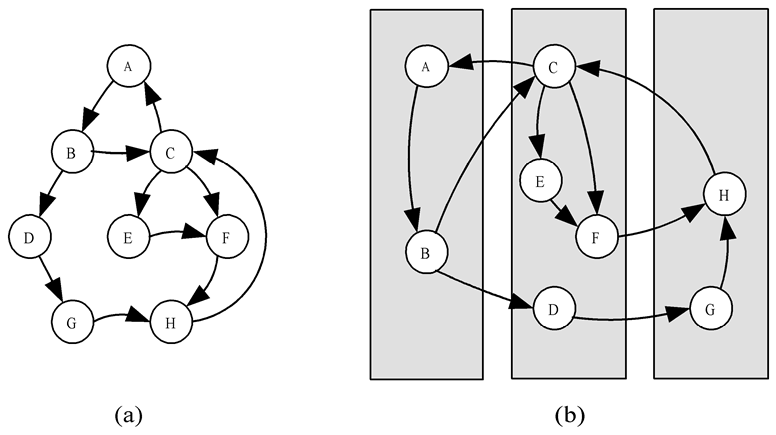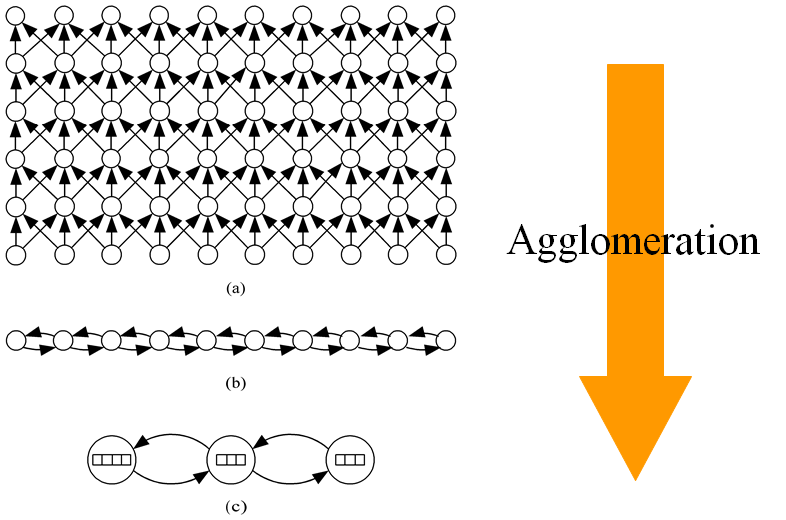• $\chi$：更新一个元素的时间
• $\lambda$：一个元素通信的时间
• $n$：结点数
• $m$：需要的迭代次数
• $p$：处理器数

• 串行执行时间：$m(n-1)\chi$
• 并行执行时间：$m\lceil(n-1)/p\rceil+2\lambda$

## # 4 Performance

### # 加速比和效率指标

$S_p = \frac{T_s}{T_p}$
• $T_s$：串行时间
• $T_p$$p$ 个进程时的并行时间（按最长时间的进程计算）
• $S_p$ or $\psi(n, p)$$p$ 个进程时的加速比 (Speedup)

$\psi(n, p) \leq \frac{\sigma(n)+\varphi(n)}{\sigma(n)+\varphi(n) / p+\kappa(n, p)}$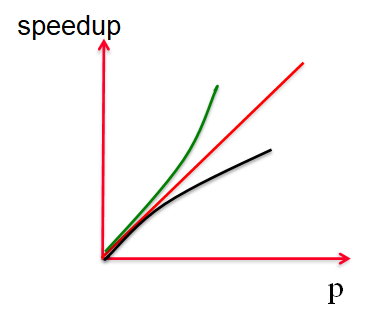$\psi(n, p) \leq \frac{\sigma(n)+\varphi(n)}{\sigma(n)+\varphi(n) / p+\kappa(n, p)}$
• $\sigma(n)$：不能被并行执行的的串行时间
• $\varphi(n)$：可以被并行执行的串行时间
• $\kappa(n, p)$：并行执行带来的通信时间

$E_p=\frac{S_p}{p}$
• $E_p$ or $\varepsilon(n, p)$：效率

$S_p$ 代入即有：

$\varepsilon(n, p) \leq \frac{\sigma(n)+\varphi(n)}{p\sigma(n)+\varphi(n)+p\kappa(n, p)}$

#### # Amdahl 定律

Amdahl 定律和 Gustafson-Barsis 定律都把通信成本放缩掉了。两个求的都是加速比，但是注意条件不一样（一个是 $f$ 一个是 $s$

$\psi(n, p) \leq \frac{\sigma(n)+\varphi(n)}{\sigma(n)+\varphi(n) / p+\kappa(n, p)}$

$f$ 为串行部分占比（占改进之前的比），即 $f=\frac{\sigma(n)}{\sigma(n)+\varphi(n)}$，有：

$\psi \leq \frac{1}{f+(1-f)/p}$

#### # Gustafson-Barsis 定律

$s$ 为串行部分占比（占改进之后的比），即 $s = \frac{\sigma(n)}{\sigma(n)+\varphi(n)/p}$，有：

$\psi \leq p + (1-p)s$

#### # Karp-Flatt Metric 指标

Amdahl 和 Gustafson-Barsis 都忽略了通信成本，会高估放大比。Karp-Flatt 从另一个角度来进行分析。

$e = \frac{\sigma(n) + \kappa(n,p)}{\sigma(n)+\varphi(n)}$

串行时间 + 通信时间 / 串行时间 + 可并行的时间

$e = \frac{1/\psi - 1/p}{1 - 1/p}$

$p$ 2 3 4 5 6 7 8
$\psi$ 1.8 2.5 3.1 3.6 4.0 4.4 4.7

$p$ 2 3 4 5 6 7 8
$\psi$ 1.9 2.6 3.2 3.7 4.1 4.5 4.7

$p$ 4 8 12
$\psi$ 3.9 6.5

? 处能否为 10？

## # 5 Message-Passing Programming

MPI 常用函数

//First MPI function called by each process
MPI_Init (&argc, &argv);

// First argument is communicator
// Number of processes returned through second argument
MPI_Comm_size (MPI_COMM_WORLD, &p);
// Process rank (in range 0, 1, …, p-1) returned through second argument
MPI_Comm_rank (MPI_COMM_WORLD, &id);

// Call after all other MPI library calls
MPI_Finalize();

// reduce 操作
int MPI_Reduce (
void *operand,      /* addr of 1st reduction element */
void *result,       /* addr of 1st reduction result, only root get result */
int count,          /* reductions to perform */
MPI_Datatype type,  /* type of elements */
MPI_Op operator,    /* reduction operator */
int root,           /* process getting result(s) */
MPI_Comm comm       /* communicator */
)
MPI_Reduce (&count, &global_count, MPI_INT, 0, MPI_COMM_WORLD);

// Benchmarking the Program
double elapsed_time;
MPI_Init (&argc, &argv);
MPI_Barrier (MPI_COMM_WORLD);
elapsed_time = - MPI_Wtime();
// ...
MPI_Reduce (…);
elapsed_time += MPI_Wtime();


### # 附 MPICH 中文教程

https://scc.ustc.edu.cn/zlsc/cxyy/200910/MPICH/

## # 6 The Sieve of Eratosthenes

int MPI_Bcast (
void *buffer,           /* Addr of 1st element */
int count,              /* # elements to broadcast */
MPI_Datatype datatype,  /* Type of elements */
int root,               /* ID of root process */
MPI_Comm comm)          /* Communicator */
MPI_Bcast (&k, 1, MPI_INT, 0, MPI_COMM_WORLD);


### # 分块算法

\begin{aligned} my\_first\_i &= i * \lfloor\frac{n}{p}\rfloor + \min(i,r) \\ my\_last\_i &= (i+1)* \lfloor\frac{n}{p}\rfloor + \min(i+1,r) - 1 \\ count &= \min(\lfloor \frac{j}{\lfloor n / p \rfloor+1}\rfloor, \lfloor \frac{j-r}{\lfloor n / p \rfloor}\rfloor) \end{aligned}

\begin{aligned} my\_first\_i &= \lfloor\frac{i*n}{p}\rfloor \\ my\_last\_i &= \lfloor\frac{(i+1)*n}{p}\rfloor - 1 \\ count &= \lfloor \frac{p(j+1)-1}{n}\rfloor \end{aligned}

### # 算法性能分析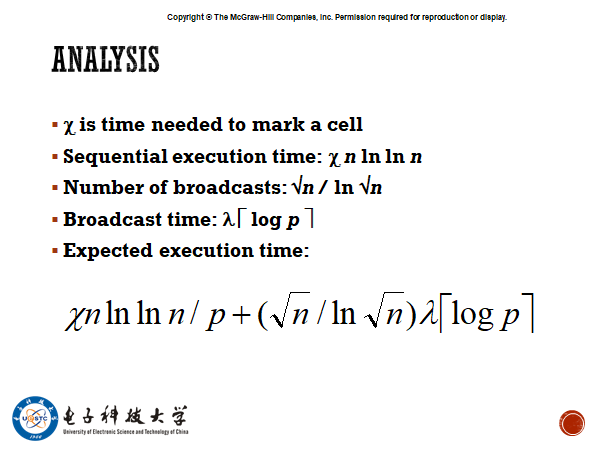## # 7 Floyd's Algorithm

Floyd 算法伪代码：

for k = 0 to n-1
for i = 0 to n-1
for j = 0 to n-1
a[i,j] = min (a[i,j], a[i,k] + a[k,j])
endfor
endfor
endfor


### # 通信### # 聚合和映射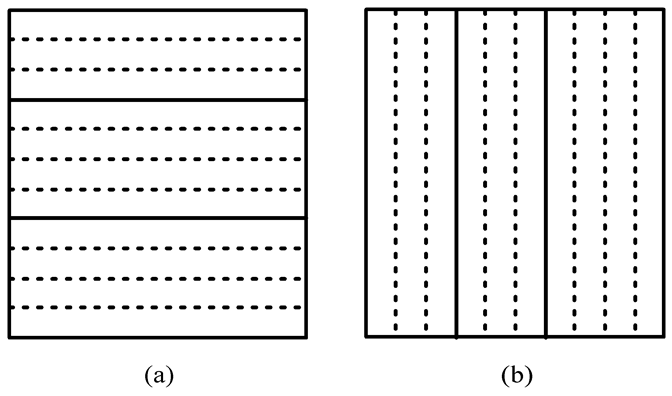### # 点对点通信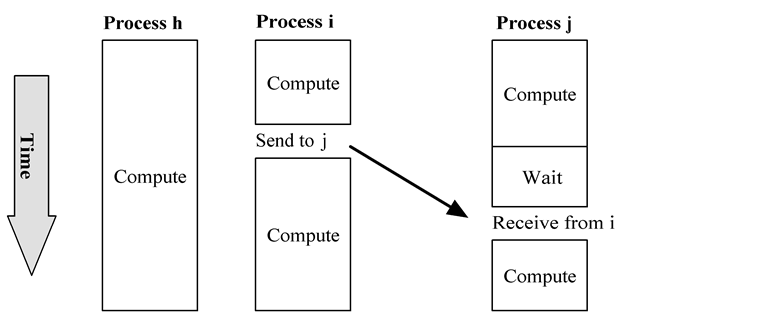int MPI_Send (
void         *message,
int           count,
MPI_Datatype  datatype,
int           dest,
int           tag,
MPI_Comm      comm
)

int MPI_Recv (
void         *message,
int           count,
MPI_Datatype  datatype,
int           source,
int           tag,
MPI_Comm      comm,
MPI_Status   *status
)


Send 和 Recv 需要约定相同的 tag，以及对方的 id (作为自己的 source/dest)。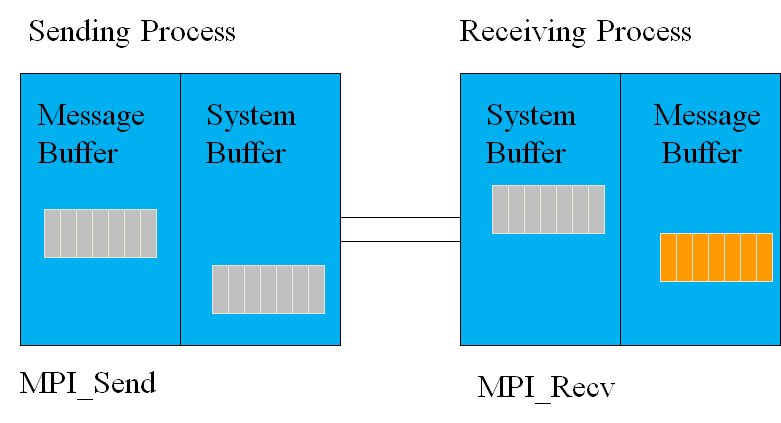• MPI_Send 函数会一直阻塞直至 message_buffer 空了。
• MPI_Recv 函数会一直阻塞直至收到消息。

#### # 死锁

if (id == 0) {
MPI_Recv (&b,...);
MPI_Send (&a,...);
c = (a + b)/2.0;
} else if (id == 1) {
MPI_Recv (&a,...);
MPI_Send (&b,...);
c = (a + b)/2.0;
}


Process 0 blocks waiting for message from 1, but 1 blocks waiting for a message from 0. Deadlock!

if (id ==0) {
MPI_Send(&a, ... 1,MPI_COMM_WORLD);
MPI_Recv(&b, ... 1, MPI_COMM_WORLD,&status);
c = (a+b)/2.0;
}else if (id ==1) {
MPI_Send(&a, ... 0,MPI_COMM_WORLD);
MPI_Recv(&b, ... 0, MPI_COMM_WORLD,&status);
c = (a+b)/2.0;
}


Both processes send before they try to receive, but they still deadlock. Why? The tags are wrong. Process 0 is trying to receive a tag of 1, but Process 1 is sending a tag of 0.

#### # Ssend

1. 双方都是先发后收，并且发的数据都很大
2. 生产者/消费者问题，且生产者生产的比消费者块

MPI 标准定义了 MPI_Ssend，保证发送会被阻塞（ssynchronous）。

#### # SendRecv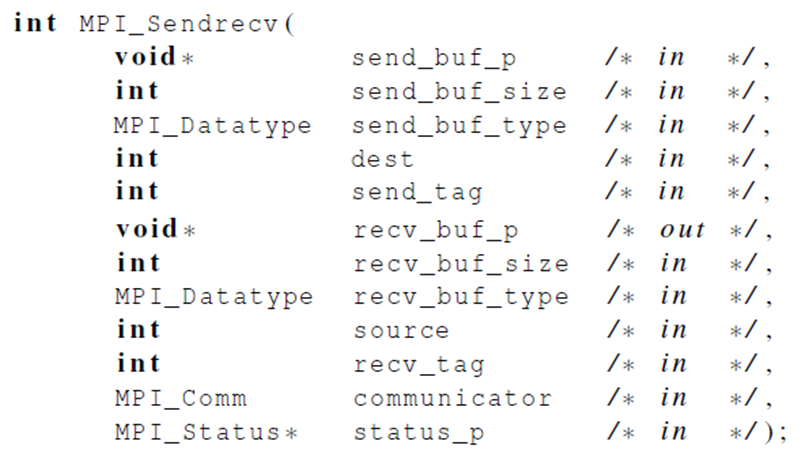### # 并行 Floyd 算法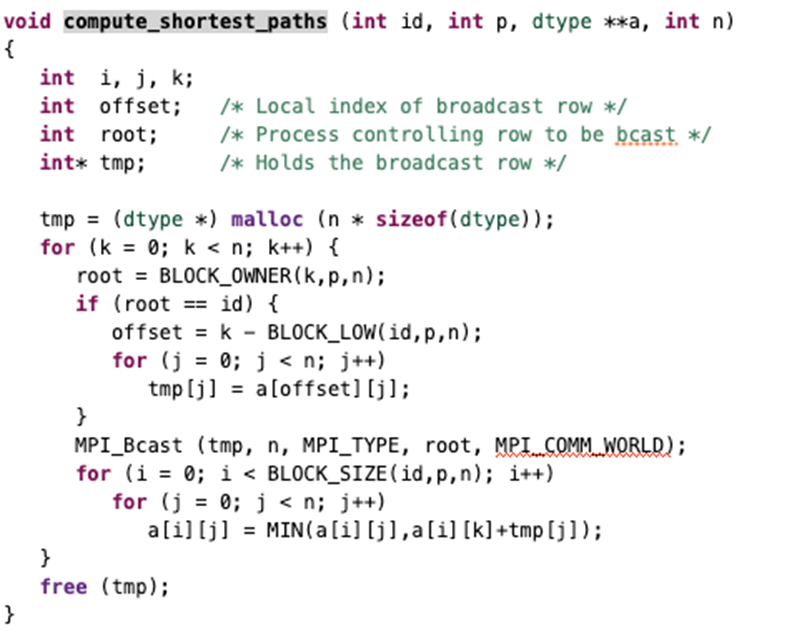• 计算时间复杂度：$\Theta(n^3/p)$
• 通信时间复杂度：$n^2 \log p$
• 执行时间（其中 $\beta$ 是显存带宽，其他变量见第三章）：
$n \lceil n / p \rceil n \chi + n \lceil \log p \rceil (\lambda + 4n / \beta)$

## # CUDA 部分

### # 结构

• Warp
• Block -- 对应一个 Streming Multiprocessors，Shared Memory
• Grid -- 对应一个 Kernel
• Device -- Global Memory

### # 代码思路

__global__ void PictureKernel(float* d_Pin, float* d_Pout, int height, int width)
{
int Row = blockIdx.y*blockDim.y + threadIdx.y;
int Col = blockIdx.x*blockDim.x + threadIdx.x;
if ((Row < height) && (Col < width)) {
d_Pout[Row*width+Col] = 2.0*d_Pin[Row*width+Col];
}
}

int main()
{
dim3 DimGrid((n-1)/16 + 1, (m-1)/16+1, 1);
dim3 DimBlock(16, 16, 1);
PictureKernel<<<DimGrid,DimBlock>>>(d_Pin, d_Pout, m, n);
}


### # Block 大小

For Matrix Multiplication using multiple blocks, should I use 8X8, 16X16 or 32X32 blocks for Fermi?

• For 8X8, we have 64 threads per Block. Since each SM can take up to 1536 threads, which translates to 24 Blocks. However, each SM can only take up to 8 Blocks, only 512 threads will go into each SM!
• For 16X16, we have 256 threads per Block. Since each SM can take up to 1536 threads, it can take up to 6 Blocks and achieve full capacity unless other resource considerations overrule.
• For 32X32, we would have 1024 threads per Block. Only one block can fit into an SM for Fermi. Using only 2/3 of the thread capacity of an SM.

### # CGMA

CGMA = 从全局内存中取一个数，多少次运算用到了这个数

CGMA 越大越好

### # Shared Memory And Threading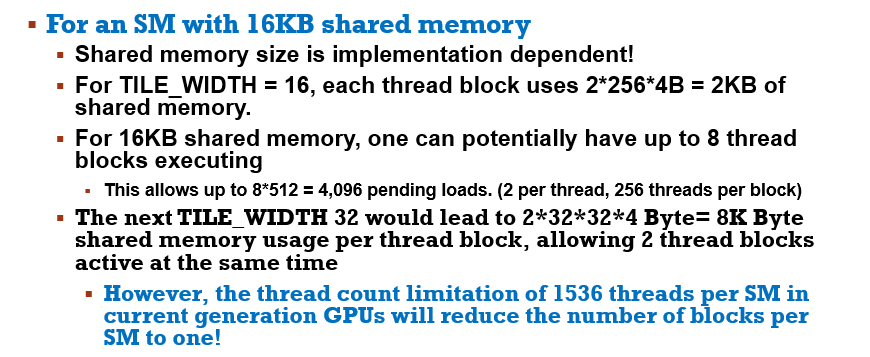## # OpenACC 部分

### # GPU 占用率

GPU Occupancy is:

• How much parallelism is running / How much parallelism the hardware could run
• 100% occupancy is not required for, nor does it guarantee best performance.
• Less than 50% occupancy is often a red flag